# Decision Making in JavaScript

Decision-Making Conditions

There can be many situations in which you might need to make some decisions based on some conditions. For example, you are a small kid and you want chocolate. But your mother/ father keeps a condition that if you complete your homework, you will get the chocolate. So, now, there is a condition for you to get the chocolate. Now you have to make a decision based on the condition that whether or not you want the chocolate.

So, I was just trying to explain what we are going to do in an abstract way. Did you notice that here, we are doing something like – ”If this, then this” type of thing?

Certainly, we are going to use the same thing to make decisions based on some conditions. We are going to use the if keyword with a condition, to decide what code should run. Let’s have a look at the syntax first -Decision Making(conditionals)

There can be many situations in which you might need to make some decisions based on some conditions. For example, you are a small kid and you want chocolate. But your mother/ father keeps a condition that if you complete your homework, you will get the chocolate. So, now, there is a condition for you to get the chocolate. Now you have to make a decision based on the condition that whether or not you want the chocolate.

So, I was just trying to explain what we are going to do in an abstract way. Did you notice that here, we are doing something like – ”If this, then this” type of thing?

### The simple if-else statements

Now, as you just say in English, if the condition is true, do this, else do that. Just like this, we are going to make use of some keywords here, like if and else, to achieve the decision-making things in JavaScript.

Certainly, we are going to use the same thing to make decisions based on some conditions. We are going to use the if keyword with a condition, to decide what code should run. Let’s have a look at the syntax first –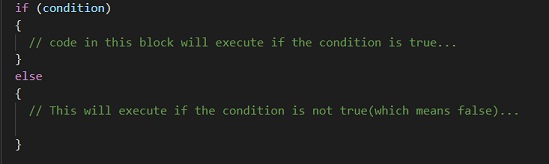one more thing is that the code in the if block will only be executed if the condition is true. If the condition is not true(which means that it is false), then the code inside the else block will execute. Let’s have a look at a simple program, through which, we can understand this in a better way. Let’s say, that we have some number stored in some variable, and we are checking whether that number is positive or negative. Well, when the number is greater than 0, it is positive, and else it is negative. So, let’s have a look at the program now –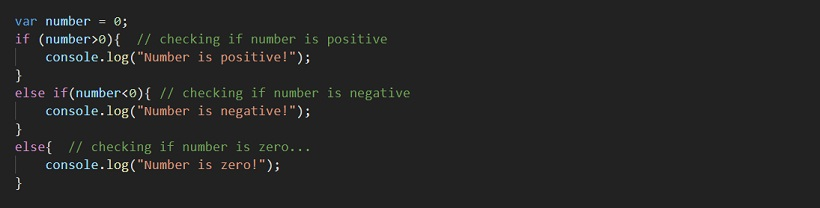If you try to have a look at the output to the console, it is something like this –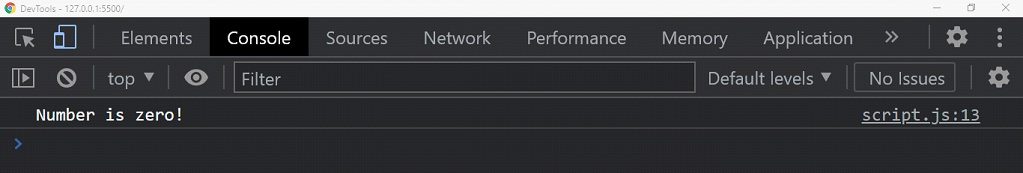You can try changing the values for the variable, and see the changes.

Well, you can see that we are doing something on the basis of some conditions. Also to mention, that the else is running, if the condition becomes false. This is like if the condition is true, do this, else do that. Note that writing the else is optional. You can have only if condition, and not write the else part, which is completely fine. But, writing the else alone does not make sense. This is like someone is saying only that – else do this, and well, it makes no sense.

Now, let’s go ahead and explore the conditional statements to more extent. Now, you might have noticed that we are writing the condition with if, and if the condition becomes false, we are just writing the else block. But here, we are not checking any conditions with the else block. But sometimes, we might need to check the conditions even after some condition becomes false.

For this, we can write the if after else as well, so we can write another condition into it. Let’s have a look at it now.

As you now know, using the if and else things, you can just simply put some condition, and do something if the condition is true. But now, let’s say that we want to check the condition, even if the condition is false. For that, we are going to use the if keyword again, after the else keyword. When you will have a look at the program, you would understand the thing.

The else if is used together to check for a new condition if the previous condition is false. This is just like the condition in the if is getting false and hence we ares running the else block, but they’re also we are checking some condition.

Now, let’s have a look at the program, which explains the else if ladder basically. In this program, we are going to do something similar to what we have already done. All we are going to do is check if the given number is less than zero, greater than zero, or zero.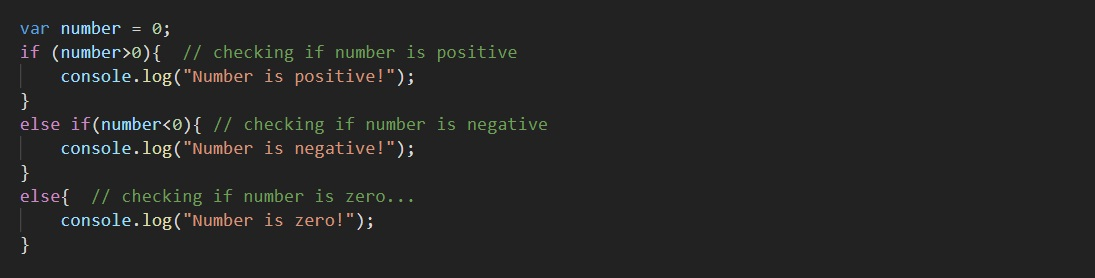As you can see, in the above program, we have used the if keyword again after the else, so that we can check some conditions again. The logic is that we are first checking whether the number is greater than zero, and if it is not, then we are checking if the number is less than zero, and else, we are printing that the number is zero. Lets now have a look at the program output –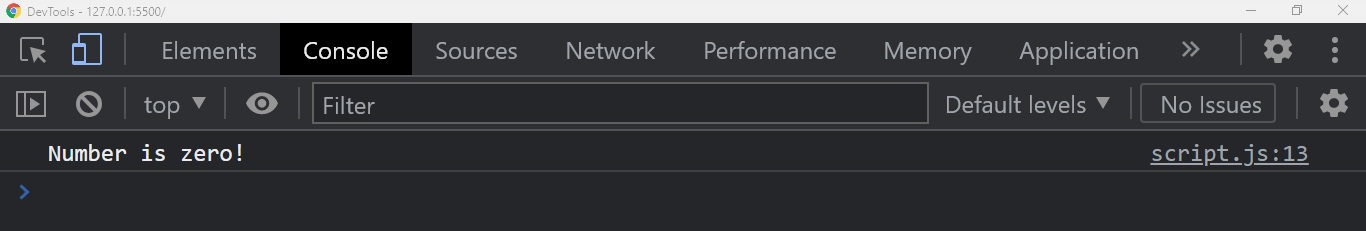### Nested if-else statements

Now, we know that we can use the if-else statements for decision making, and also we can have if after else so that we can check the condition after some condition is false. But, in some situations, it might happen that we might need to write if inside an if statement, we would call this nesting, so, now we are going to have a look at this. We often do nesting for a bunch of situations, like if there are many conditions to be checked, etc.
The below code shows how you can nest the conditions.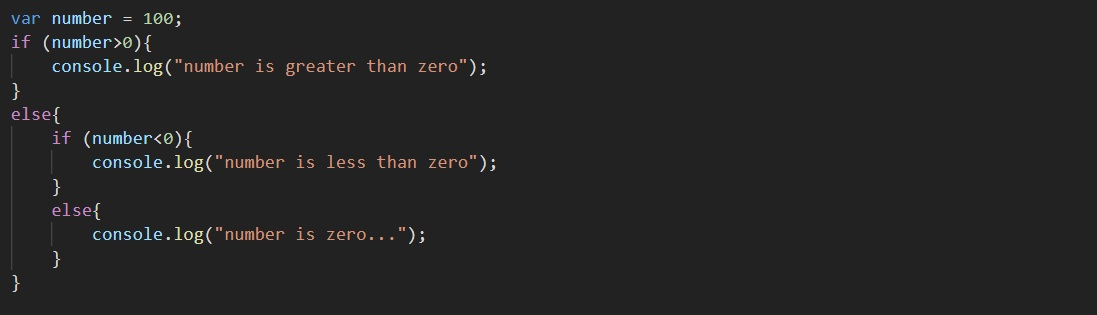As you can see, we have written an if inside an else, which is nesting here. You can try more complex examples of nesting conditional statements. But this gives us an idea of what nesting means. If you run to the console for the output, the output is something like this –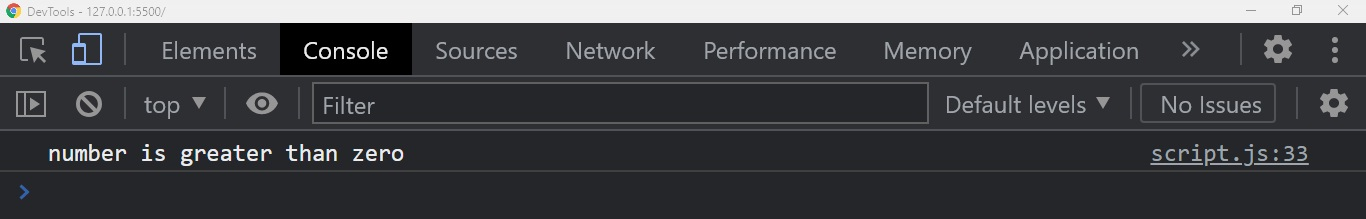As you can see, the output comes out to be relevant. You can nest the statements, as and when needed. You can try out some different examples, to get familiar with the concept. The concept of decision-making is very very important, and we often are required to use it.